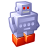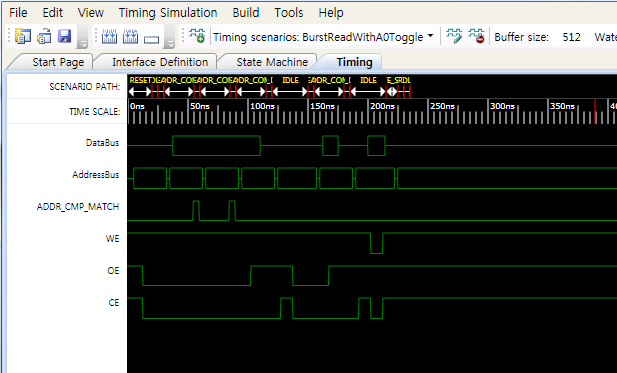cancel
Showing results for
Did you mean:

USB superspeed peripheralsNew Contributor

I want to write data to eMMC using gpiftoStorage example file

GPIF is set to Async SRAM interface.The MCU (DSP) is programmed as follows, but does not write data from the FX3S to the eMMC memory.

eMMC memory clock and data does not change.

Please. Tell me what went wrong in the MCU (DSP) program.

/* Break the request data into bytes. */

*(volatile Uint16 *)(0x280000 + (0x0000 * 2)) = 0x0600;

*(volatile Uint16 *)(0x280000 + (0x0000 * 2)) = 0x0000;

*(volatile Uint16 *)(0x280000 + (0x0000 * 2)) = 0x0100;

*(volatile Uint16 *)(0x280000 + (0x0000 * 2)) = 0x0000;

DELAY_US(1000);

/* 512byte Write */

int s = 0;

for(s=0;s<512;s++)

{

DELAY_US(1);

*(volatile Uint16 *)(0x280000 + (0x0000 * 2)) = s;

DELAY_US(1);

}